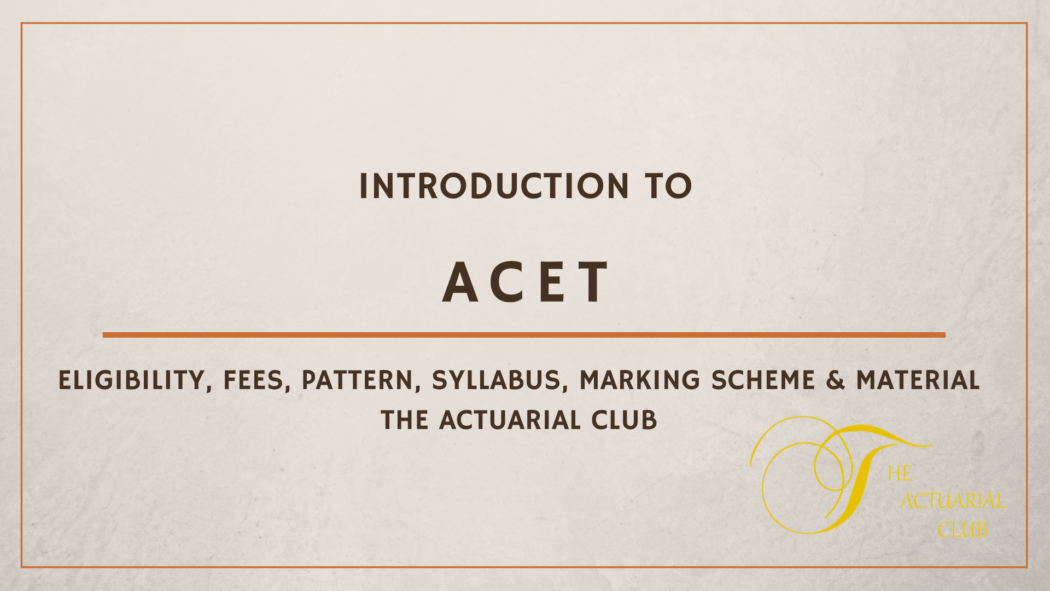# A Guide to Actuarial Common Entrance Test

A guide to preparing for Actuarial Common Entrance Test (ACET)

The actuarial common entrance test is popularly referred to as ACET. The exam is administered three times per year and is offered by the Institute of Actuaries of India. It is a computer-based exam that consists of MCQ questions which should be completed in a certain period. The learners have a second chance to sit for the examination if they do not pass it on their first attempt.

An ACET exam is administered to qualified students. Only registered students get a confirmation call that invites them to the examination. The institute sends links with details on how to register, pay for the exam, and upload your photos.

Contents

## Eligibility

To be eligible for ACET exams, the learner must have passed the 10+2 examination including English. People with similar qualifications and those with superior education qualifications can be allowed to sit for the exams. Also, students who have studied mathematics at a higher level could be allowed to take the examination.

Also, students that have pursued an engineering course, company secretaries, accountants, and graduates who hold a degree in mathematics and statistics could be allowed to take the exams.

## Registration fee

Students aspiring to take the exams need to pay a non-refundable Rs 3,000 as examination registration fee. They will then receive a sample question paper upon registration. Learners will get access to a marking scheme and an online exam.

### ACET exam

The first step is to become a member of the Institute, i.e. the candidate has to pass an entrance exam. IAI had started conducting its entrance exam Actuarial Common Entrance Test (ACET) in January 2012. This exam has undergone many changes in its structure from the year 2012.

The exam features MCQ type questions and lasts for about 3 hours. It consists of 70 MCQ questions with one correct answer.

The current ACET pattern is as follows:

### Marks allocation

1. 45 questions carry 1 mark each
2. 20 questions carry 2 marks each
3. 05 questions carry 3 marks each

### Marking scheme

To pass the ACET examination, the learners will be expected to score at least 50% from the following distribution

• Statistics 30 marks
• Logical reasoning 10 marks
• Mathematics 30 marks English 15
• Data interpretation 15 marks

## ACET Exam syllabus

Anyone preparing to sit for ACET exam must study the following subjects

Mathematics – here, the learner will be expected to cover the following:

• Algebra
• Vectors
• Notational and standard functions
• Integration
• Differentiation
• Numerical methods
• Matrices

In statistics, the learners will be expected to study the following:

• Types of data- histogram, bar charts, statistical diagrams, boxplot, mode, median, mean stem-leaf
• Measures of spread- Skewness, variance, standard deviation and interquartile range,
• Probability- rules of probability(Basic)
• Advanced probability- conditional probabilities and tree diagram
• Discrete random variables- coefficient of skewness, variance, and standard deviation mean medium and mode, definitions and probabilities
• Continues random variables- coefficient of skewness, variance, standard deviation mean medium and mode, definitions and probabilities
• Discrete distributions – Poisson distribution, binomial, Bernoulli, Discrete uniform
• Correlation- correlation coefficient, covariance, scatter plots,
• Regression

The candidate should study the following topics while preparing for the exam in English

• Usage of English and grammar
• Correlations in sentences
• Filling the blank spaces
• Reverse analogies and analogies
• Cloze passage
• Jumbled paragraph
• Verbal reasoning
• Summary questions
• Judgment/inference /facts

Other areas

Data interpretation- here, the data comes in the form of charts, graphs, and tables. The candidate is tested on how they can interpret school data and answer the subsequent questions. Other diagrams that could be used are Venn diagrams, pie charts, line charts, caselets, and bar graphs as well as column graphs.

The learner must also prepare in the following topics

• Calendar
• Letter series and numbers
• Cubes
• Calendars
• Binary logic
• Cubes
• Logical matching
• Seating arrangement
• Logical connectives
• Blood relations
• Syllogism
• Logical sequence

## Books for ACET exam